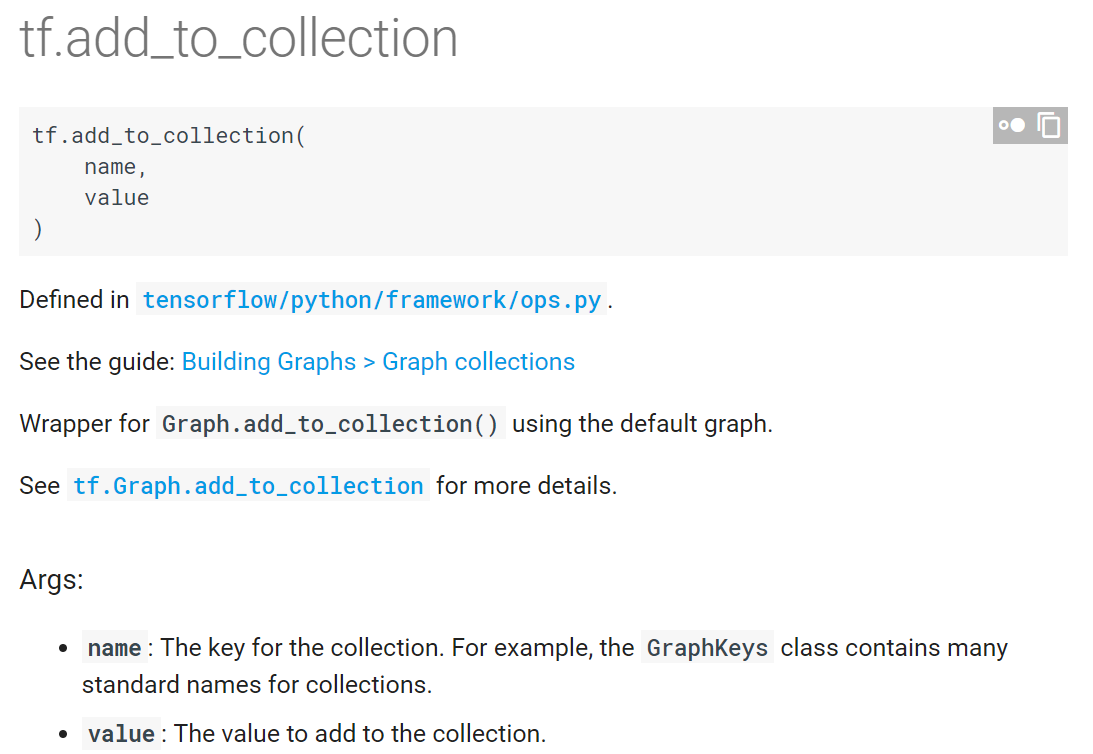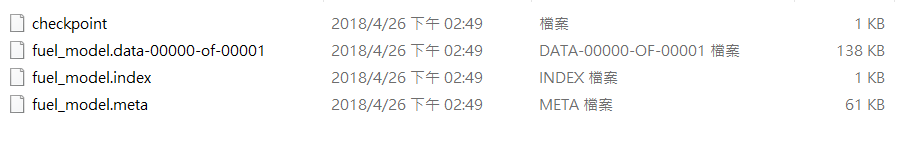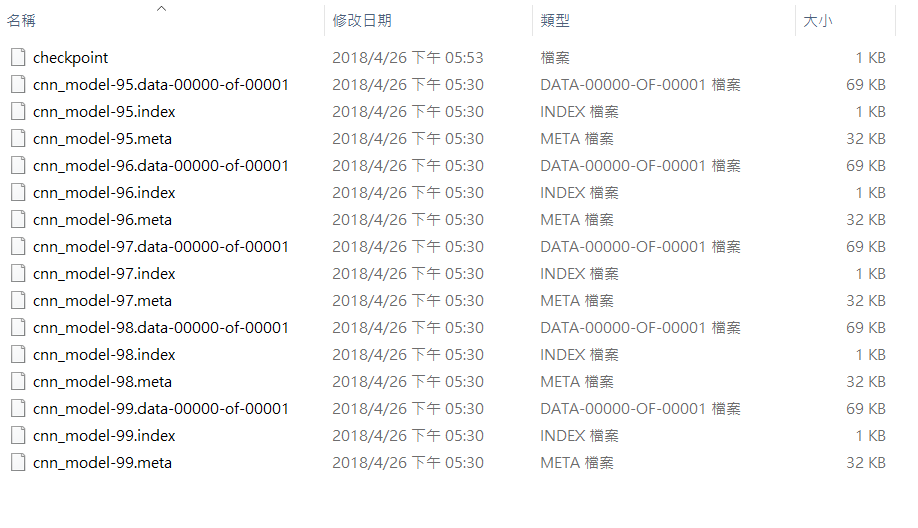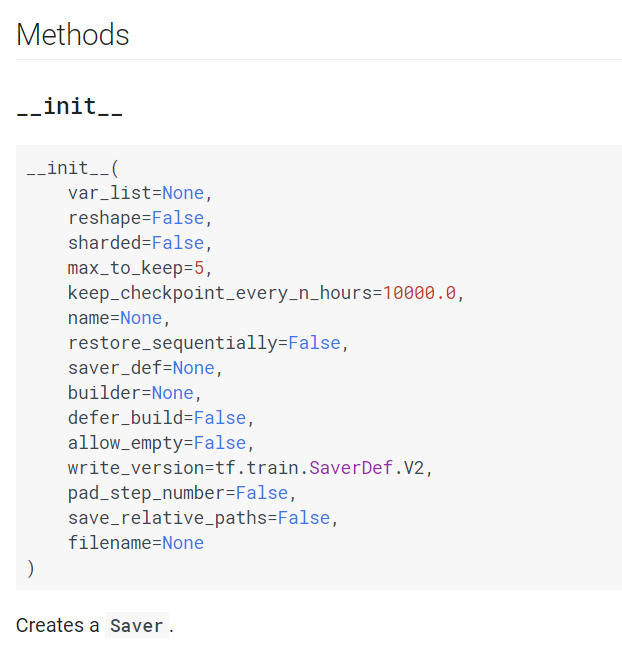## tf.train.Saver

tf.train.Saver 就是 TensorFlow 所提供的存檔工具。

# -*- coding: utf-8 -*-
"""

"""

import tensorflow as tf

# 建立文件名隊列
filename_queue = tf.train.string_input_producer([filename], num_epochs=None)

# 數據讀取器

# 數據解析
img_features = tf.parse_single_example(
serialized_example,
features={ 'Label'    : tf.FixedLenFeature([], tf.int64),
'image_raw': tf.FixedLenFeature([], tf.string), })

image = tf.decode_raw(img_features['image_raw'], tf.uint8)
image = tf.reshape(image, [42, 42])

label = tf.cast(img_features['Label'], tf.int64)

# 依序批次輸出 / 隨機批次輸出
# tf.train.batch / tf.train.shuffle_batch
image_batch, label_batch =tf.train.shuffle_batch(
[image, label],
batch_size=batch_size,
capacity=10000 + 3 * batch_size,
min_after_dequeue=1000)

return image_batch, label_batch

###############
# 以下為主程式 #

# tfrecord 檔案位置
filename = './py_Train.tfrecords'

# batch 可以自由設定
batch_size = 256

# 0-9共10個類別，請根據自己的資料修改
Label_size = 10

# 轉換陣列的形狀
image_batch_train = tf.reshape(image_batch, [-1, 42*42])

# 把 Label 轉換成獨熱編碼
label_batch_train = tf.one_hot(label_batch, Label_size)

# W 和 b 就是我們要訓練的對象
W = tf.Variable(tf.zeros([42*42, Label_size]))
b = tf.Variable(tf.zeros([Label_size]))

# 我們的影像資料，會透過 x 變數來輸入
x = tf.placeholder(tf.float32, [None, 42*42])

# 這是參數預測的結果
y = tf.nn.softmax(tf.matmul(x, W) + b)

# 這是每張影像的正確標籤
y_ = tf.placeholder(tf.float32, [None, 10])

# 計算最小交叉熵
cross_entropy = tf.reduce_mean(tf.nn.softmax_cross_entropy_with_logits_v2(labels=y_, logits=y))

# 使用梯度下降法來找最佳解

# 計算預測正確率
correct_prediction = tf.equal(tf.argmax(y, 1), tf.argmax(y_, 1))
accuracy = tf.reduce_mean(tf.cast(correct_prediction, tf.float32))

####################################################
####################################################
# 新增的內容在這邊 #

# 計算 y 向量的最大值
y_pred = tf.argmax(y, 1)

# 建立 tf.train.Saver 物件
saver = tf.train.Saver()

# 將輸入與輸出值加入集合

####################################################
####################################################

with tf.Session() as sess:
# 初始化是必要的動作
sess.run(tf.global_variables_initializer())
sess.run(tf.local_variables_initializer())

# 建立執行緒協調器
coord = tf.train.Coordinator()

# 啟動文件隊列，開始讀取文件

# 迭代 100 次，看看訓練的成果
for count in range(100):
# 這邊開始讀取資料
image_data, label_data = sess.run([image_batch_train, label_batch_train])

# 送資料進去訓練
sess.run(train_step, feed_dict={x: image_data, y_: label_data})

# 這裡是結果展示區，每 10 次迭代後，把最新的正確率顯示出來
if count % 10 == 0:
train_accuracy = accuracy.eval(feed_dict={x: image_data, y_: label_data})
print('Iter %d, accuracy %4.2f%%' % (count, train_accuracy*100))

# 結束後記得把文件名隊列關掉
coord.request_stop()

####################################################
# 這裡也是新增的內容 #

# 存檔路徑 #
save_path = './model/test_model'

# 把整張計算圖存檔
spath = saver.save(sess, save_path)
print("Model saved in file: %s" % spath)
####################################################

####################################################
####################################################
# 新增的內容在這邊 #

# 計算 y 向量的最大值
y_pred = tf.argmax(y, 1)

# 建立 tf.train.Saver 物件
saver = tf.train.Saver()

# 將輸入與輸出值加入集合

####################################################
##################################################### 分別存到 x 集合與 y 集合

###

# 通通存到 A 集合
tf.add_to_collection('A', y_pred)

####################################################
# 這裡也是新增的內容 #

# 存檔路徑 #
save_path = './model/test_model'

# 把整張計算圖存檔
spath = saver.save(sess, save_path)
print("Model saved in file: %s" % spath)

####################################################

## tf.train.restore# -*- coding: utf-8 -*-
"""

"""

import cv2
import tensorflow as tf

with tf.Session() as sess:

##################################################
save_path = "./model/test_model.meta"

# 使用 import_meta_graph 載入計算圖
saver = tf.train.import_meta_graph(save_path)

# 使用 restore 重建計算圖
saver.restore(sess, "./model/test_model")

# 取出集合內的值
x = tf.get_collection("input")
y = tf.get_collection("output")

##################################################

# 讀一張影像

# 辨識影像，並印出結果
result = sess.run(y, feed_dict = {x: img.reshape((-1, 42*42))})
print(result)


### 1. 若存檔時使用同一個集合名稱，範例如下：

# 通通存到 A 集合
tf.add_to_collection('A', y_pred)

# 取出集合內的值
x = tf.get_collection("A")
y = tf.get_collection("A")

### 2. 若是在即時辨識系統中，建議把重建的步驟擺在第一步：

# 以下為建議的寫法
with tf.Session() as sess:

##################################################

# tf.train.restore...
# 重建計算圖很費時，請放在無限迴圈的外面

##################################################

while True:
# 即時影像辨識流程...

# 辨識影像，並印出結果
result = sess.run(y, feed_dict = {x: img.reshape((-1, 42*42))})
print(result)

# 以下為不建議的寫法
while True:

# 即時影像辨識流程...
# ...

with tf.Session() as sess:
# 重建計算圖
# tf.train.restore...

# 辨識影像，並印出結果
result = sess.run(y, feed_dict = {x: img.reshape((-1, 42*42))})
print(result)


## 模型訓練進階

global_step 參數會在存檔時接續在檔名的末端，使得我們可以大量存檔，以下為 Saver.save 的調用方法：

spath = saver.save(sess, save_path, global_step=count)tf.train.Saver 物件的預設值是保留最近五次的訓練結果，預設值的修改在建立物件時就可以指定，import tensorflow as tf

# 建立 tf.train.Saver 物件
saver = tf.train.Saver()

# 存檔路徑 #
save_path = './model/test_model'

# 狀態指定
iscontinue = 1

with tf.Session() as sess:
# 初始化
sess.run(tf.global_variables_initializer())
sess.run(tf.local_variables_initializer())

coord = tf.train.Coordinator()

if not iscontinue:
# 迭代 100 次，看看訓練的成果
for count in range(100):
# 讀取資料
image_data, label_data = sess.run([image_batch_train, label_batch_train])

# 訓練
sess.run(train_step, feed_dict={x: image_data, y_: label_data})

# 結果展示
if count % 10 == 0:
train_accuracy = accuracy.eval(feed_dict={x: image_data, y_: label_data})
print('Iter %d, accuracy %4.2f%%' % (count, train_accuracy*100))

# 存檔
spath = saver.save(sess, save_path, global_step=count)
print("Model saved in file: %s" % spath)

else:
# 重建 model #
last_ckp = tf.train.latest_checkpoint("./model")
saver = tf.train.import_meta_graph(last_ckp+'.meta')
saver.restore(sess, last_ckp)

# 延續舊有資料繼續迭代 100 次
for count in range(100, 200):
# 讀取資料
image_data, label_data = sess.run([image_batch_train, label_batch_train])

# 訓練
sess.run(train_step, feed_dict={x: image_data, y_: label_data})

# 結果展示
if count % 10 == 0:
train_accuracy = accuracy.eval(feed_dict={x: image_data, y_: label_data})
print('Iter %d, accuracy %4.2f%%' % (count, train_accuracy*100))

spath = saver.save(sess, save_path, global_step=count)
print("Model saved in file: %s" % spath)

# 關掉文件名隊列
coord.request_stop()
coord.join(threads)

【Python】TensorFlow學習筆記(六)：卷積的那些小事